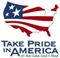Scientific Investigations Report 2007–5050

U.S. GEOLOGICAL SURVEY
Scientific Investigations Report 2007–5050
Version 1.1, April 2010

## Conversion Factors and Datums

### Conversion Factors

Multiply By To obtain
acre 0.004047 square kilometer (km2)
acre-foot (acre-ft) 1,233 cubic meter (m3)
acre-foot per year (acre-ft/yr) 1,233 cubic meter per year (m3/yr)
cubic foot (ft3) 0.02832 cubic meter (m3)
cubic foot per second (ft3/s) 0.02832 cubic meter per second (m3/s)
cubic foot per second per mile [(ft3/s)/mi] 0.0176 cubic meter per second per kilometer [(m3/s)/km]
foot (ft) 0.3048 meter (m)
foot per day (ft/d) 0.3048 meter per day (m/d)
foot per mile (ft/mi) 0.1894 meter per kilometer (m/km)
foot squared per day (ft2/d) 0.09290 meter squared per day (m2/d)
gallon (gal) 3.785 liter (L)
gallon per day per foot [(gal/d)/ft] 0.01242 cubic meter per day per meter [(m3/d)/m]
gallon per minute (gal/min) 0.06309 liter per second (L/s)
inch (in.) 2.54 centimeter (cm)
inch per year (in/yr) 25.4 millimeter per year (mm/yr)
mile (mi) 1.609 kilometer (km)
million gallons (Mgal) 3,785 cubic meter (m3)
million gallons per day (Mgal/d) 0.04381 cubic meter per second (m3/s)
square foot (ft2) 0.09290 square meter (m2)
square mile (mi2) 2.590 square kilometer (km2)

Temperature in degrees Fahrenheit (°F) may be converted to degrees Celsius (°C) as follows:

°C = (°F-32)/1.8.

### Datums

Horizontal coordinate information is referenced to the North American Datum of 1927 (NAD 27).”

Vertical coordinate information is referenced to the National Geodetic Vertical Datum of 1929 (NGVD 29). In this report, “altitude” refers to the distance above the vertical datum.

*Transmissivity: The standard unit for transmissivity is cubic foot per day per square foot times foot of aquifer thickness [(ft3/d)/ft2]ft. In this report, the mathematically reduced form, foot squared per day (ft2/d), is used for convenience.U.S. Department of the Interior | U.S. Geological Survey